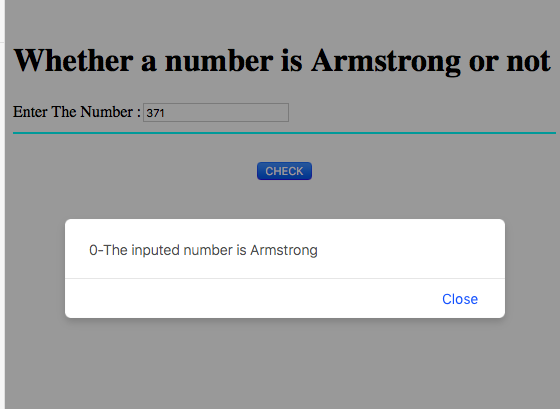# JavaScript code to check number is Armstrong or not

In this JavaScript code, we are going to check whether a given number is Armstrong number or not.
Submitted by Aleesha Ali, on March 28, 2018

## Armstrong number

A number is said to be Armstrong when its value is equal to its sum of cube of digits.

Example:

```    Input:
Number: 153

Output:
It is a prime number

Explanation:
153 = (1*1*1) + (5*5*5) + (3*3*3) = 1+125+27 = 153
Here, number (153) is equal to its sum of the digits cube (153).
```

### JavaScript code to check whether a Number is Armstrong or Not

```<script>
function Armstrong()
{
number = Number(document.getElementById("N").value);

flag = number;
while(number > 0)
{
remainder = number%10;
number = parseInt(number/10);
}

{
}
else
{
window.alert("-The inputed number is not Armstrong");
}
}
</script>
```

Calling JavaScript function in HTML (HTML, JS Code)

```<html>
<script>
function Armstrong()
{
number = Number(document.getElementById("N").value);

flag = number;
while(number > 0)
{
remainder = number%10;
number = parseInt(number/10);
}

{
}
else
{
window.alert("-The inputed number is not Armstrong");
}
}
</script>
<body>
<br>
<h1>Whether a number is Armstrong or not</h1>
Enter The Number :<input type="text" name="n" id = "N"/>
<hr color="cyan">
<br>
<center><button onClick="Armstrong()">CHECK</button>
</body>
</html>
```

Output